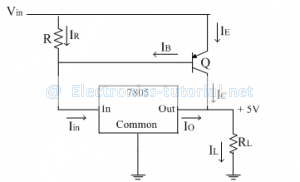# Current Booster

If the output current requirement is as high as 10A, then it is convenient to boost a regulator output current with the help of external circuit.
The current boosting can be achieved by using external transistor connected in parallel with regulator IC. Thus the output current of 78XX regulator which is 1A can be boosted.
The IC 7805 regulator with current booster is shown below.Analysis:
Applying KCL at the output side
IL=IC+IO
IL=βIB+IO
But IB+IR=Iin
∴IB=Iin-IR
For IC 7805, neglecting the common terminal current, we get
Io=Iin
∴IB=IO-IR

The current through resistance R is given as
IR=VBE/R
∴IB=IO-VBE/R
Substituting this IB in the load current equation
IL=β[IO-VBE/R]+IO
IL=βIO-βVBE/R+IO
Thus the current boosting is achieved which depends on the value of 'β' and maximum output current of the regulator IC.

Limitations of linear voltage regulators:
1)Low efficiency.
2)For high input voltages efficiency decreases.
3)Power dissipated in control element (series pass transistor) is more.
4)Due to low input frequency (50 Hz) filter capacitances of large values are required.